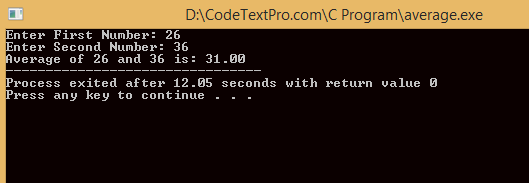# C Program to find the Average of two numbers || How to find Average of numbers

## C Program to find the average of two numbers

In this C program, you will learn how to find the average number between two numbers.

### Example #1: Average of Two Numbers

#include <stdio.h>
int main()
{
int num1, num2;
float avg;

printf("Enter First Number: ");
scanf("%d",&num1);
printf("Enter Second Number: ");
scanf("%d",&num2);

avg= (float)(num1+num2)/2;

//%.2f is used for displaying output up to two decimal places

printf("Average of %d and %d is: %.2f",num1,num2,avg);

return 0;
}

### Output of Program:C Program to find the Average of two numbers

## Find the average of two numbers using function

In this program, we have to create a user-defined function average() for the calculation of average. The numbers entered by the user are passed to this function during the function call.

### Example #2: C Program to find the Average of Two Number Using Function

#include <stdio.h>
float average(int a, int b){
return (float)(a+b)/2;
}
int main()
{
int num1, num2;
float avg;

printf("Enter first number: ");
scanf("%d",&num1);
printf("Enter second number: ");
scanf("%d",&num2);

avg = average(num1, num2);

//%.2f is used for displaying output upto two decimal places
printf("Average of %d and %d is: %.2f",num1,num2,avg);

return 0;

}

Output of Program:C program to find the average of two numbers using function